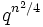# Classification of abelian subgroups of maximum order in unipotent upper-triangular matrix groups

(diff) ← Older revision | Latest revision (diff) | Newer revision → (diff)
Let$p$ be a prime number, and let$q = p^r$ be a power of$p$. Let$U(n,q)$ denote the group of upper-triangular unipotent$n \times n$ matrices over the field of$q$ elements. Note that$U(n,q)$ is a$p$-Sylow subgroup of the general linear group$GL(n,q)$.
Then the abelian subgroups of maximum order in$U(n,q)$ are given as follows:
• For odd$n$, there are two such groups, both of them elementary Abelian of order$q^{(n^2 - 1)/4}$. The first group is given as the group of all upper-triangular matrices with$1$s on the diagonal, and zeroes elsewhere except in the top right$(n-1)/2 \times (n+1)/2$ rectangle. The second group is given as the group of all upper-triangular matrices with$1$s on the diagonal, and zeroes elsewhere except in the top right$(n+1)/2 \times (n-1)/2$ rectangle. Both these subgroups are normal: they are related via an outer automorphism which is the composite of conjugation by the antidiagonal matrix and the transpose-inverse map.
• For even$n$, there is exactly one Abelian subgroup of maximum order. This is elementary Abelian of order$q^{n^2/4}$. It is given as the group of upper-triangular matrices with$1$s on the diagonal and zeroes elsewhere except in the top right$n \times n$ square.